# PROBABILITY - Concept with Example.

INTRODUCTION

The theory of probability has been originated from the game of chance and gambling. In old days, gamblers used to gamble in a gambling house with a die to win the amount fixed among themselves. They were always desirous to get the prescribed number on the upper face of a die when it was thrown on a board. Shakuni of Mahabharat was perhaps one of them. People started to study the subject of probability from the middle of seventeenth century. The mathematicians Huygens, Pascal Fermat and Bernoulli contributed a lot to this branch of Mathematics. A.N. Kolmogorow proposed the set theoretic model to the theory of probability. Probability gives us a measure of likelihood that something will happen. However probability can never predict the number of times that an occurrence actually happens. But being able to quantify the likely occurrence of an event is important because most of the decisions that affect our daily lives are based on likelihoods and not on absolute certainties.

DEFINITIONS

Experiment :

An action or operation resulting in two or more well defined outcomes. e.g. tossing a coin, throwing a die, drawing a card from a pack of well shuffled playing cards etc.

Sample space :

A set S that consists of all possible outcomes of a random experiment is called a sample space and each outcome is called a sample point. Often, there will be more than one sample space that can describe outcomes of an experiment, but there is usually only one that will provide the most information.

e.g. in an experiment of "throwing a die", following sample spaces are possible : (i) {even number, odd number}
(ii) {a number less than 3, a number equal to 3, a number greater than 3}
(iii) {1,2,3,4,5,6}

Here 3rd sample space is the one which provides most information.

If a sample space has a finite number of points it is called finite sample space and if it has an infinite number of points, it is called infinite sample space. e.g. (i) "in a toss of coin" either a head (H) or tail (T) comes up, therefore sample space of this experiment is S = {H,T} which is a finite sample space. (ii) "Selecting a number from the set of natural numbers", sample space of this experiment is S = {1,2,3,4,......} which is an infinite sample space.

E v e n t:

An event is defined as an occurrence or situation, for

example

(i) in a toss of a coin, it shows head,

(ii) scoring a six on the throw of a die,

(iii) winning the first prize in a raffle,

(iv) being dealt a hand of four cards which are all clubs.

In every case it is set of some or all possible outcomes of the experiment. Therefore event (A) is subset

of sample space (S). If outcome of an experiment is an element of A we say that event A has occurred.

• An event consisting of a single point of S is called a simple or elementary event.

•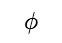is called impossible event and S (sample space) is called sure event.

Compound Event :

If an event has more than one sample points it is called Compound Event. If A & B are two given events then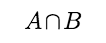is called compound event and is denoted byor AB or A & B.

Complement of an event :

The set of all outcomes which are in S but not in A is called the complement of the event A.

Mutually Exclusive Events :

1. Two events are said to be Mutually Exclusive (or disjoint or incompatible) if the occurrence of one precludes (rules out) the simultaneous occurrence of the other. If A & B are two mutually exclusive events then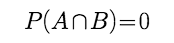Consider, for example, choosing numbers at random from the set {3, 4, 5, 6, 7, 8, 9, 10, 11, 12}

If, Event A is the selection of a prime number,

Event B is the selection of an odd number,

Event C is the selection of an even number,

then A and C are mutually exclusive as none of the numbers in this set is both prime and even. But A and B are not mutually exclusive as some numbers are both prime and odd (viz. 3, 5, 7, 11).

Equally Likely Events :

Events are said to be Equally Likely when each event is as likely to occur as any other event. Note that the term 'at random' or 'randomly' means that all possibilities are equally likely.

Exhaustive Events :

Events A,B,C........ N are said to be Exhaustive Events if no event outside this set can result as an outcome of an experiment. For example, if A & B are two events defined on a sample space S and A & B are exhaustive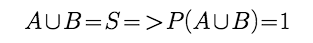Example :

A coin is tossed three times, consider the following events.
B : 'exactly one head appears'
C : 'at least two heads appear'
Do they form a set of mutually exclusive and exhaustive events ?

Solution :

The sample space of the experiment is
S = {HHH, HHT, HTH, THH, HTT, THT, TTH, TTT}
Events A, B and C are given by
A = {TTT}
B = {HTT, THT, TTH}
C = {HHT, HTH, THH, HHH}

Now,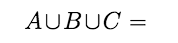{TTT, HTT,THT,TTH,HHT,HTH,THH,HHH} = S

Therefore A,B and C are exhaustive events. Also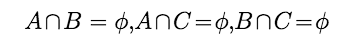Therefore, the events are pair-wise disjoint, i.e., they are mutually exclusive. Hence, A,B and C form a set of mutually exclusive and exhaustive events.

Example :

Consider the experiment in which a coin tossed repeatedly until a head comes up. Describe the Sample Space?

Solution :

In the experiment head may come up on the first toss, or the first toss, or the 2nd toss, or the 3rd toss and so on
Hence, the desired sample space is S = {H, TH, TTH, TTTH, TTTTH,...}

CLASSICAL DEFINITION OF PROBABILITY :

If n represents the total number of equally likely, mutually exclusive and exhaustive outcomes of an experiment and m of them are favourable to the happening of the event A, then the probability of happening of the event A is given by P(A) = m/n. There are (n–m) outcomes which are favorable to the event that A does not happen.

'The event A does not happen' is denoted by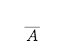(and is read as 'not A')

Thus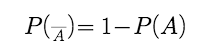Point to remember: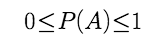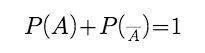This relationship is most useful in the 'at least one' type of problems.

If x cases are favourable to A & y cases are favourable to not A then P(A) = x/x+y and P(not A) = y/x+y.We say that Odds In Favour Of A are x: y & Odds Against A are y : x

Empirical probability

The probability that you would hit the bull's-eye on a dartboard with one throw of a dart would depend on how much you had practised, how much natural talent for playing darts you had, how tired you were, how good a dart you were using etc. all of which are impossible to quantify. A method which can be adopted in the example given above is to throw the dart several times (each throw is a trial) and count the number of times you hit the bull's-eye (a success) and the number of times you miss (a failure). Then an empirical value of the probability that you hit the bull's-eye with any one throw is

number of successes/number of successes + number of failures .

If the number of throws is small, this does not give a particular good estimate but for a large number of throws the result is more reliable.
When the probability of the occurrence of an event A cannot be worked out exactly, an empirical value can be found by adopting the approach described above, that is :

1. (i)  making a large number of trials (i.e. set up an experiment in which the event may, or may not, occur and note the outcome),

2. (ii)  counting the number of times the event does occur, i.e. the number of successes,

3. (iii)  calculating the value of number of successes /  number of trials (i.e. successes + failures) = r/ n

Example :

A coin is tossed successively three times. Find the probability of getting exactly one head or two heads.

Solution :

Let S be the sample space and E be the event of getting exactly one head or exactly two heads, then

S = {HHH, HHT, HTH, THH, TTH, THT, HTT, TTT}. and E = {HHT, HTH, THH, HTT, THT, TTH}
n(E) = 6 and n(S) = 8.

Now required probability, P(E) = n(E) /n(S)=  6/8 = 3/4

Example :

Words are formed with the letters of the word PEACE. Find the probability that 2 E’s come together.

Solution :

Total number of words which can be formed with the letters P, E, A, C, E = 5!/2! = 60

Numberofwordsinwhich2E’scometogether 4! = 24

Required probability = 24/60 = 2/5

Example :

From a group of 10 persons consisting of 5 lawyers, 3 doctors and 2 engineers, four persons are selected at random. The probability that the selection contains at least one of each category is

Solution :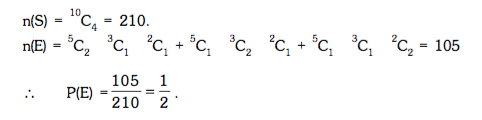Post By : Devendra Yadav 29 Apr, 2019 1991 views Maths Probability